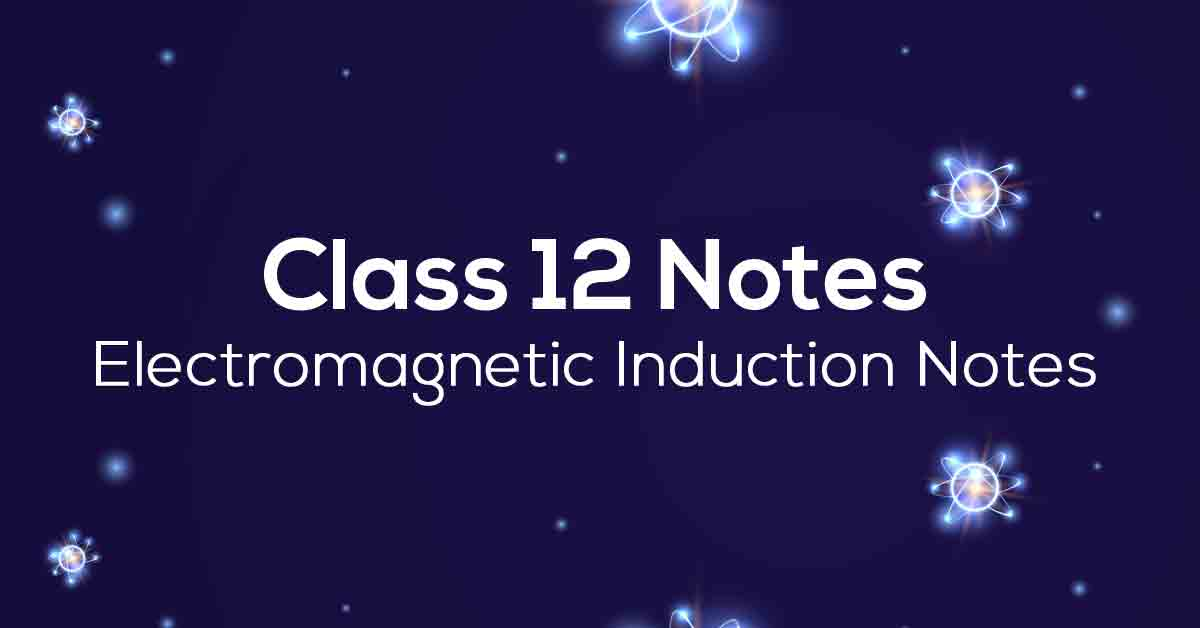Class 12th Physics Electromagnetic Induction NCERT Notes CBSE 2023

# Electromagnetic Induction Class 12 Notes# Chapter 6 Electromagnetic Induction

Here we will cover AC generators, magnetic flux, the energy density in magnetic fields, and the experiments of Faraday and Henry. Vidyakul provides the Grade 12 NCERT notes with over 1000 practice questions and 6 reference books. We provide guided notes developed by NCERT to help students achieve good test scores. Students can get Electromagnetic Induction notes from Grade 12 with the following unlimited practice questions and answers.

### Points to Remember

Below we have provided some of the important points to remember for this chapter to ace your exam:

• According to Lenz’s law, a change in magnetic flux tends to produce a current that opposes the polarity of the induced emf. This is indicated by the negative sign in Faraday’s law.

• Eddy current: Changing magnetic fields can set up current loops in any conductor body. Such currents are eddy currents. They dissipate electrical energy as heat.

• By virtue of electromagnetic induction converts mechanical energy to electrical energy in the AC generator.

• The induced emf across the ends is ε=Blv when a metal rod of length (l) is normal to a uniform magnetic field (B) and moves with a velocity (v) perpendicular to the field.

• The magnetic flux through a surface of area A placed in a uniform magnetic field B is defined as ϕB=B⋅A=BA cosθ where θ is the angle between B and A.

### Topics and Sub-topics

 Topic Name Topic Name Topic Name The Experiments Of Faraday And Henry Lenz’S Law And Conservation Of Energy Eddy Currents Magnetic Flux Motional Electromotive Force Inductance Faraday’S Law Of Induction Energy Consideration: A Quantitative Study Mutual Inductance

### Few Important Questions

• Two coils X and Y are placed in a circuit such that when the current changes by 2 A in coil X the magnetic flux changes by 0.4 Wb in Y. What is the value of mutual inductance of the coils?

The value of the mutual inductance of the coils is 0.2 H.

• A conducting loop of area 5.0 cm2 is placed in a magnetic field which varies sinusoidally with time as B = B0 sin ωt where B0 = 0.20 T and ω = 300 s−1. The normal to the coil makes an angle of 600 with the direction of the field. Find the maximum emf induced in the coil.

The maximum emf induced in the coil is 0.015 V.

• A metal wheel with 8 metallic spokes, each 40 cm long, is rotated at a speed of 120 rev/min in a plane perpendicular to earth’s magnetic field of 0.3 × 10−4 T. Find the magnitude of the induced emf between the axis and the rim of the wheel?

3 × 10−5 V emf will be induced between the axis and the rim of the wheel.

### Practice Questions

1. If the current carried by the solenoid changes steadily from 4 A to 7 A in 1 s, what is the induced emf in the loop? The solenoid has 30 turns per cm and has a small loop of area 4 cm2 placed inside the solenoid normal to its axis.

2. What is the emf developed across the cut if the velocity of the loop is 3 cm s–1 in a direction normal to the loop, longer side, and shorter side? For how long does the induced voltage last in each case if the rectangular wire loop with dimensions of 15 cm and 10 cm with a small cut is moving out of a region of the uniform magnetic field of magnitude 0.5

3. If the length of the long metallic rod is 3.0 m and is rotated with an angular frequency of 700 rad s–1 about an axis normal to the rod passing through its one end. Calculate the emf developed between the center and the ring. A circular metallic ring is in contact with the other end of the rod. A constant and uniform magnetic field of 0.10 T parallel to the axis exists everywhere.